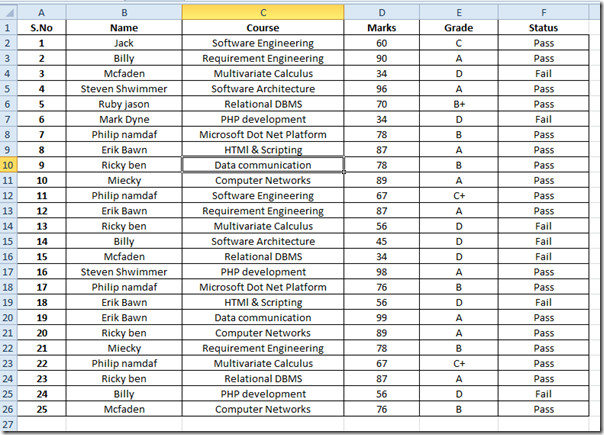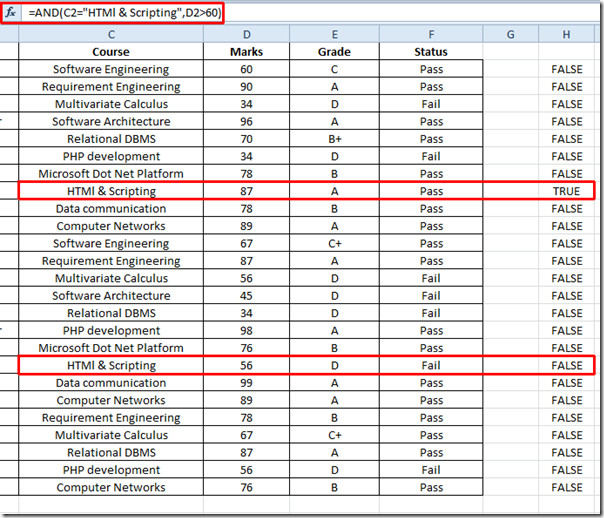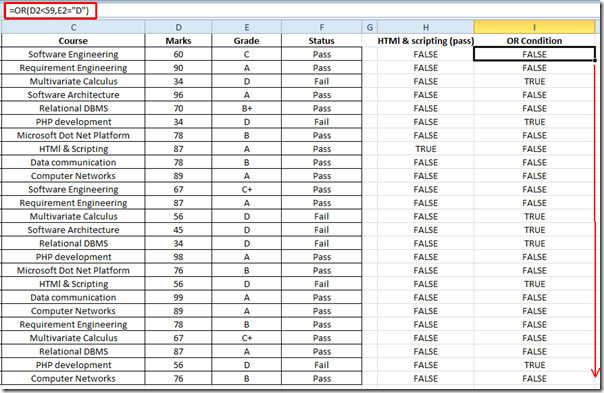# Excel 2010: Basic Logical Functions

Using logical functions provided by Excel 2010, we could apply and evaluate the specific rationale on the data. The most commonly used logical functions are AND, OR, and NOT. They take values as arguments to apply a simple logic over them and yield TRUE and FALSE result. The logical function helps users in situations where they want to categorize the value into groups, so that further complex functions can be easily performed. For example, if you want to check two different values that belong to different fields against a condition, you can use the logical function to check where the required condition is met. This may assist you in filtering out unwanted values and in focusing on only those values that you require. Additionally, Excel 2010 lets you use the multiple logical functions to check required conditions for the data set in one go. In what follows, we will demonstrate the usage of AND & OR logical functions.

To get started, launch Excel 2010 spreadsheet, containing the data set on which you want to apply logical functions. For illustration, we will apply AND & OR logical functions in a spreadsheet containing fields; Name, Course, Marks, Grade, and Status, as shown in the screenshot below.Now we will apply logical operations over different fields. Since we want to find out that how many students registered HTML & Scripting course and secured 60+ marks, we use AND function. This function returns TRUE if both specified conditions are met.

If student studied HTML & scripting and secured marks greater than 60, only then the condition is said to be TRUE.

The syntax of the function is;

=AND(logical1, logical2…)

We will write it as;

=AND(C2=”HTMl & Scripting”,D2>60)

C2 and D2 in the function arguments are the location of the cell from where we started searching. The output True can bee seen in the screenshot below only where both conditions have met. Conversely FALSE will appear where any of the condition is not met.Now we are going to find out the row against either Marks less than 60 or Status is fail. For this we will write OR logical function as;

=OR(D2<59,E2=”D”)

Where D2 and E2 are the locations of the cells in Marks and Grade field respectively. As TRUE can be seen against all the values in either field Marks or Grade where marks are less than 60 or grade is D.You can also check out previously reviewed Excel function; DOLLAR, SUMPRODUCT, SUMIF, COUNTIF, VLOOKUP, HLOOKUP ,PMT, and LEN.

1.antireaver says:

I need to get a logical functions formula that can carry 4 conditions. All i keep seeing is for 2 alone. Please help.

Thanks

2.ccg001 says:

I am trying to determine if the value in one column (A5555) matches or is equal to the value of ONLY the first five characters only in a another column (A5555-drod@home.com). Is there a way to limit the logic check to only a limited number of characters in a cell?

3.DM says:

=IF(AND(B1=Q1;B1=R1);B1;”some other value”)

This formula contains an if which returns the value of B1 in case of True, otherwise it returns “some other value” (when the statement is false).

The AND checks whether B1 is equal to Q1 AND B1 is equal to R1. If that is true, then the if statement is true, otherwise it is false.

Hope this helps.

4.Bcarternc says:

I’m trying to get a value using “if/or” together.  For example. If B1 Contains the value in cell q1 & r1 then  b1 (meaning that I want the value of b1 to appear int he cell formula.) Is this possible?

This site uses Akismet to reduce spam. Learn how your comment data is processed.